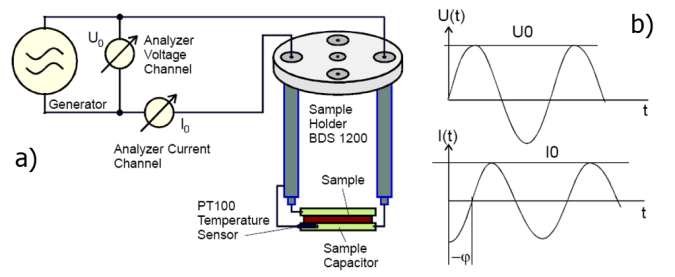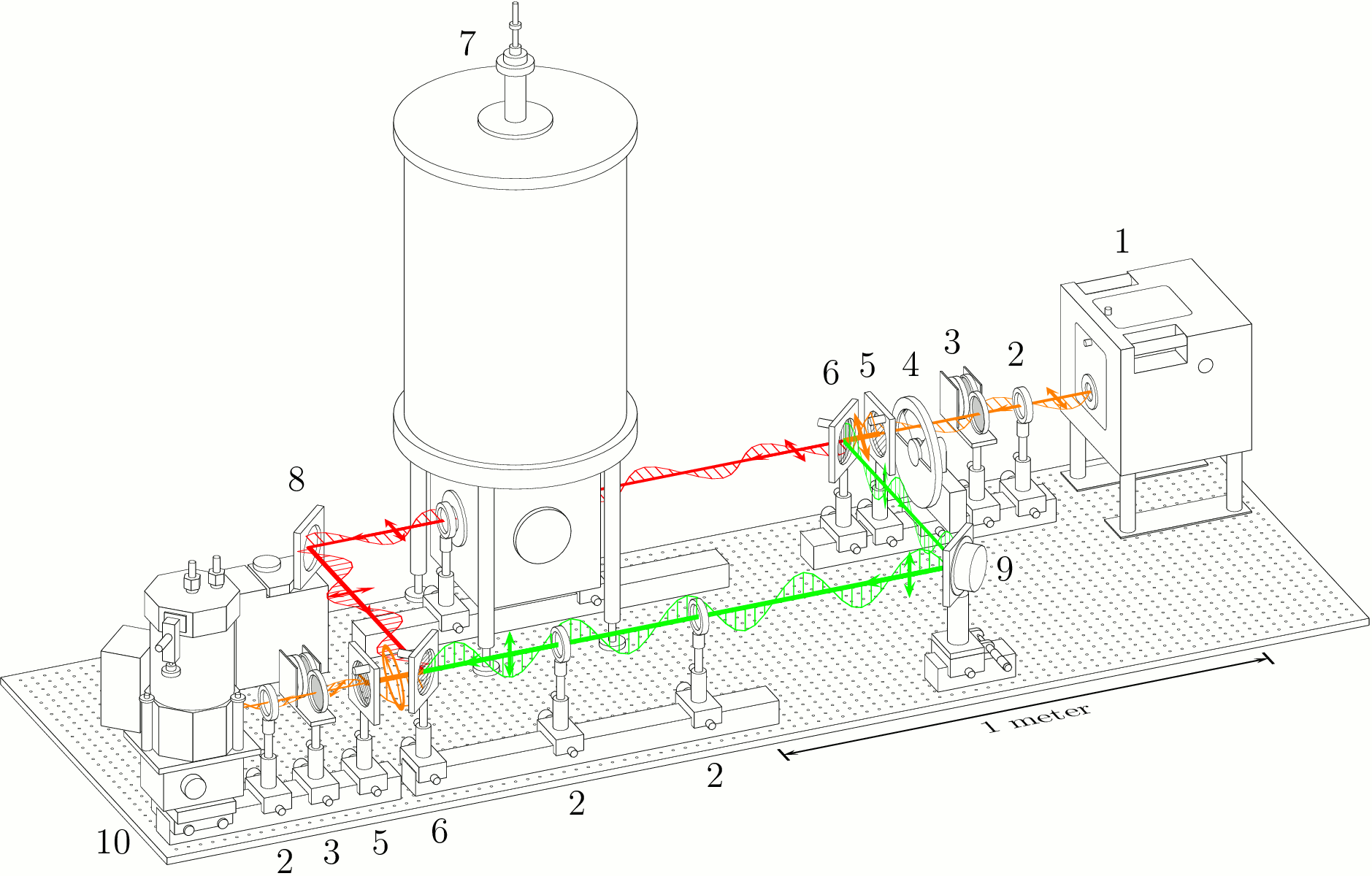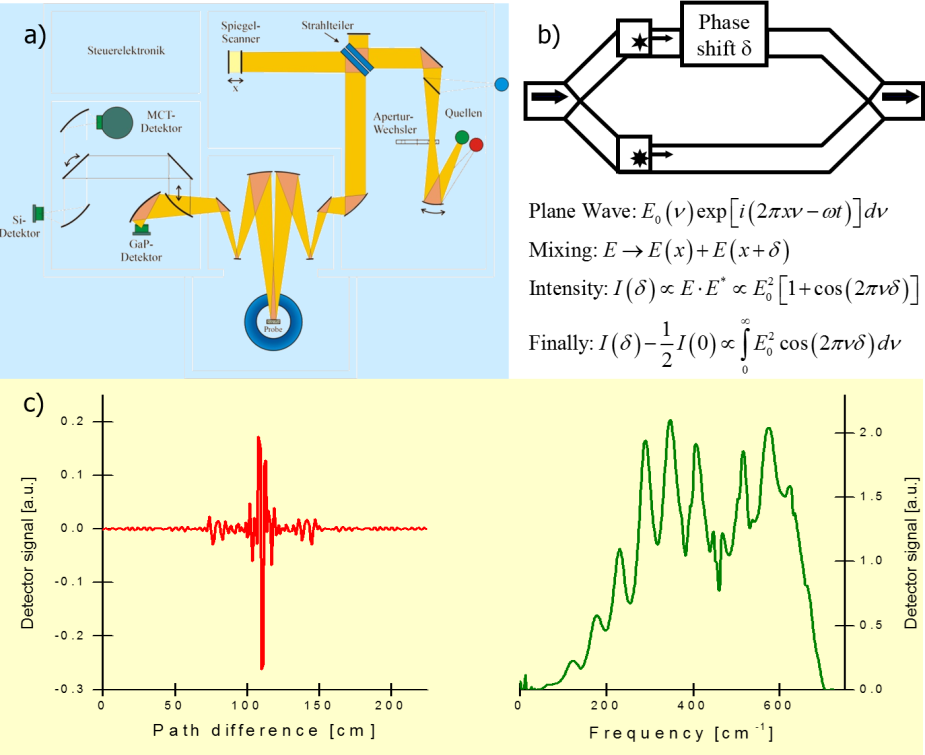# Research activities

We study the interaction of electromagnetic waves with condensed matter in a broad frequency range between 10-2 and 10+14 Hz. While in the frequency regime between 10+11 and 10+14 Hz , phonon interaction with electromagnetic waves can be studied, molecular and collective dipolar fluctuations, charge transport and electric polarization effects take place below 10+11 Hz. All these microscopic mechanisms are covered by dielectric dispersion and absorption phenomena, which determine the dielectric properties of the material under study. The broadband dielectric spectroscopy therefore is a powerful method to reveal fundamental properties of condensed matter such as quantum spin hall effect and half-integer quantum hall effect in topological insulators, charge dynamics at the metal-insulator transition, electron spin resonance in ferro- and antiferromagnetic media, magneto electric and magneto dielectric effect in multiferroic materials linked to the so called electromagnons, dynamic properties of magneto electric multifferoic phase transitions, transversal and longitudinal resonances and quasiparticle relaxation rates in superconductors, negative refraction in metamaterials, anomalous refraction in photonic crystals, ect.

In order to study all these phenomena in a broad frequency range between visible light and statics, several distinct experimental techniques are utilized.

## Experimental techniques

In the low frequency range (ν < 1 MHz) the sample in form of a capacitor is investigated. The LRC analyzer obtains the amplitude and phase shift of the current through the sample. The sample capacitance C which is proportional to the dielectric permittivity ε1+iε2 is obtained from the complex impedance as C= 1/iωZ. This method is called Dielectric Spectroscopy.Fig. a) Principle of dielectric measurement [Novocontrol GmbH] and b) Amplitude and phase shift between ac voltage, U0sinωt, and ac current, I0sin(ωt+φ), through the sample.

At higher frequencies (1 MHz < ν < 10 GHz) the cable effects are getting important. Therefore, the sample is placed as an end-element of the coaxial cable. The reflectance (r) in the cable is measured and it is given by:

r=(Z-Z0)/(Z+Z0)

Here Z = 1/ iωC is the complex impedance of the sample, and Z0 = 50 Ω is the wave-impedance of the cable.

In the terahertz frequency range a BWO-type spectrometer is used, which allows measurement of the complex transmission and reflection coefficients as a function of external parameters like magnetic fields or temperature. Using Fresnel optical equations permittivity, conductivity or refractive index is obtained without additional assumptions.Scheme of a submillimeter Mach-Zender interferometer. The main arm with the sample is in the upper left of the scheme (red rays), while the reference arm is shown in the right bottom by the green rays. Short arrows indicate the polarization of the beams. 1 - backward wave oscillator (BWO), 2 - lenses, 3 - attenuators, 4 - chopper, 5 - wire grid polarizers, 6 - wire grid beam splitters, 7 - Oxfort cryomagnet, 8 - movable mirror, 9 - oscillating mirror (phae modulator), 10 - bolometer.

In the infrared and optical frequency ranges (1Thz < ν < 1000THz) a Fourier-Transform technique is utilized and the reflectance is the basic property which is obtained in experiment. Within this technique instead of spectral scan a measurement of the broadband source is carried out as a function of phase shift (δ,x) between the halves of the beam (see figures). The inverse Fourier-Transform of the measured signal corresponds directly to the frequency spectrum of interest. To get the optical parameters the Kramers-Kronig transformation has to be applied to the spectra. The combination with the transmittance data from the terahertz range substantially improves the accuracy of the Kramers-Kronig transformation.Fig. a) Scheme of the Fourier-Transform Spectrometer. [F.Mayr], b) Principle of the Fourier-Transform measurement, c) Typical measured signal (Interferogramm, left) and the fourier-transformed spectrum (right). [F. Mayr]